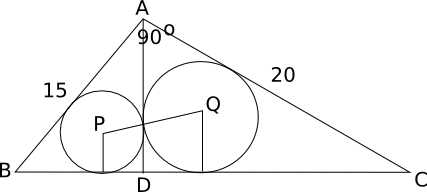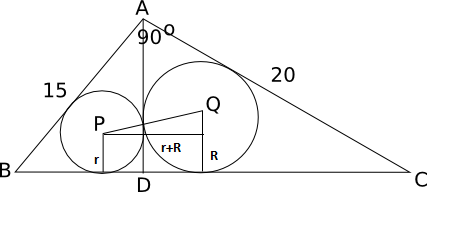Question 43

# In the following figure, ACB is a right-angled triangle. AD is the altitude. Circles are inscribed within the triangle ACD and triangle BCD. P and Q are the centers of the circles. The distance PQ isThe length of AB is 15 m and AC is 20 m

SolutionBy Pythagoras theorem we get BC = 25 . Let BD = x;Triangle ABD is similar to triangle CBA => AD/15 = x/20 and also triangle ADC is similar to triangle ACB=> AD/20 = (25-x)/15. From the 2 equations, we get x = 9 and DC = 16

We know that AREA = (semi perimeter ) * inradius

For triangle ABD, Area = 1/2 x BD X AD = 1/2 x 12 x 9 = 54 and semi perimeter = (15 + 9 + 12)/2 = 18. On using the above equation we get, inradius, r = 3.

PQ = R + r = 7 cm

### View Video Solution

• All Quant CAT Formulas and shortcuts PDF
• 20+ CAT previous papers with solutions PDF

##### AJAY

2 years ago

wrong ans, final ans will be sq rt 50 for PQ. by right angle traingle formed by PQS.

##### Rupak

2 years, 10 months ago

thank you ..it was very helpful..

OR